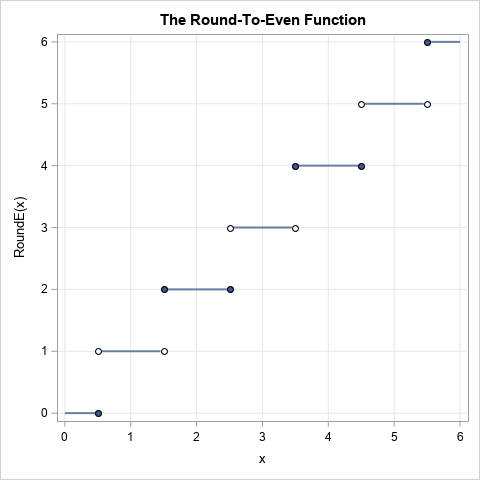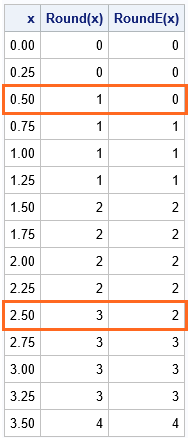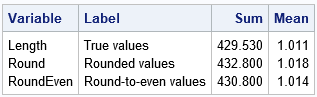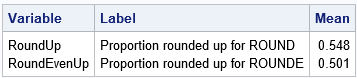In grade school, students learn how to round numbers to the nearest integer. In later years, students learn variations, such as rounding up and rounding down by using the greatest integer function and least integer function, respectively. My sister, who is an engineer, learned a rounding method that rounds half-integers to the nearest even number. This method is called the round-to-even method. (Other names include the round-half-to-even method, the round-ties-to-even method, and "bankers' rounding.") When people first encounter the round-to-even method, they are often confused. Why would anyone use such a strange rounding scheme? This article describes the round-to-even method, explains why it is useful, and shows how to use SAS software to apply the round-to-even method.### What is the round-to-even method of rounding?

The round-to-even method is used in engineering, finance, and computer science to reduce bias when you use rounded numbers to estimate sums and averages. The round-to-even method works like this:

• If the difference between the number and the nearest integer is less than 0.5, round to the nearest integer. This familiar rule is used by many rounding methods.
• If the difference between the number and the nearest integer is exactly 0.5, look at the integer part of the number. If the integer part is EVEN, round towards zero. If the integer part of the number is ODD, round away from zero. In either case, the rounded number is an even integer.

All rounding functions are discontinuous step functions that map the real numbers onto the integers. The graph of the round-to-even function is shown to the right.

I intentionally use the phrase "round away from zero" instead of "round up" because you can apply the rounding to positive or negative numbers. If you round the number -2.5 away from zero, you get -3. If you round the number -2.5 towards zero, you get -2. However, for simplicity, the remainder of the article uses positive numbers.

### Examples of the round-to-even method of roundingFor the number 2.5, which integer is closest to it? Well, it's a tie: 2.5 is a "half-integer" that is just as close to 2 as it is to 3. So which integer should you choose as THE rounded value? The traditional rounding method rounds the midpoint between two integers away from zero, so 2.5 is traditionally rounded to 3. This produces a systematic bias: all half-integers are rounded away from zero. This fact leads to biased estimates when you use the rounded data in an analysis.

To reduce this systematic bias, you can use the round-to-even method, which rounds some half-integers away from zero and others towards zero. For the round-to-even method, the number 2.5 rounds down to 2, whereas the number 3.5 rounds up to 4.

The table at the right shows some decimal values and the results of rounding the values under the standard method and the round-to-even method. The second column (Round(x)) shows the result of the traditional rounding method where all half-integers are rounded away from 0. The third column (RoundE(x)) is the round-to-even method that rounds half-integers to the nearest even integer. The red boxes indicate numbers for which the Round and RoundE functions produce different answers. Notice that for the round-to-even method, 50% of the half-integers round towards 0 and 50% round away from 0. In the table, 0.5 and 2.5 are rounded down by the round-to-even method, whereas 1.5 and 3.5 are rounded up.

### Why use the round-to-even method of rounding?

The main reason to use the round-to-even method is to avoid systematic bias when calculating with rounded numbers. One application involves mental arithmetic. If you want to estimate the sum (or mean) of a list of numbers, you can mentally round the numbers to the nearest integer and add up the numbers in your head. The round-to-even method helps to avoid bias in the estimate, especially if many of the values are half-integers.

Most computers use the round-to-even method for numerical computations. The round-to-even method has been a part of the IEEE standards for rounding since 1985.

### How to use the round-to-even method in SAS?

SAS software supports the ROUND function for standard rounding of numbers and the ROUNDE function ('E' for 'even') for round-to-even rounding. For example, the following DATA step produces the table that is shown earlier in this article:

```data Seq; keep x Round RoundEven; label Round = "Round(x)" RoundEven="RoundE(x)"; do x = 0 to 3.5 by 0.25; Round = round(x); /* traditional: half-integers rounded away from 0 */ RoundEven = rounde(x); /* round half-integers to the nearest even integer */ output; end; run;   proc print data=Seq noobs label; run;```

### An application: Estimate the average length of lengths with SAS

Although the previous sections discuss rounding values like 0.5 to the nearest integer, the same ideas apply when you round to the nearest tenth, hundredth, thousandth, etc. The next example rounds values to the nearest tenth. Values like 0.95, 1.05, 1.15, etc., are equidistant from the nearest tenth and can be rounded up or down, depending on the rounding method you choose. In SAS, you can use an optional argument to the ROUND and ROUNDE functions to specify the unit to which you want to round. For example, the expression ROUND(x, 0.1) rounds x to the nearest tenth.

An example in the SAS documentation for PROC UNIVARIATE contains the effective channel length (in microns) for 425 transistors from "Lot 1" of a production facility. In the data set, the lengths are recorded to two decimal places. What would be the impact on statistical measurements if the engineer had been lazy and decided to round the measurements to one decimal place, rather than typing all those extra digits?

The following SAS DATA step rounds the data to one decimal place (0.1 microns) by using the ROUND and ROUNDE functions. The call to PROC MEANS computes the mean and sum of the unrounded and rounded values. For the full-precision data, the estimate of the mean length is 1.011 microns. If you round the data by using the standard rounding method, the estimate shoots up to 1.018, which overestimates the average. In contrast, if you round the data by using the round-to-even method, the estimate is 1.014, which is closer to the average of the unrounded numbers (less biased). Similarly, the Sum column shows that the sum of the round-to-even values is much closer to the sum of the unrounded values.

```/* round real data to the nearest 0.1 unit */ data rounding; set Channel1; Round = round(Length, 0.1); /* traditional: round to nearest tenth */ RoundEven = rounde(Length, 0.1); /* use round-to-even method to round to nearest tenth */ /* create a binary indicator variable: Was x rounded up or down? */ RoundUp = (Round > Length); /* 1 if rounded up; 0 if rounded down */ RoundEvenUp = (RoundEven > Length); run;   proc means data=rounding sum mean ndec=3; label Length="True values" Round ="Rounded values" RoundEven="Round-to-even values"; var Length Round RoundEven; run;```As mentioned earlier, when you use the traditional rounding method, you introduce a bias every time you encounter a "half-unit" datum such as 0.95, 1.05, or 1.15. For this real data, you can count how many data were rounded up versus rounded down by each method. To get an unbiased result, you should round up the half-unit data about as often as you round down. The following call to PROC MEANS shows the proportion of data that are rounded up and rounded down by each method. The output shows that about 55% of the data are rounded up by the traditional rounding method, whereas a more equitable 50.1% of the values are rounded up by the round-to-even method.

```proc means data=rounding mean ndec=3; label RoundUp = "Proportion rounded up for ROUND" RoundEvenUp= "Proportion rounded up for ROUNDE"; var RoundUp RoundEvenUp; run;```This example illustrates a general truism: The round-to-even method is a less biased way to round data.

### Summary

This article explains the round-to-even method of rounding. This method is not universally taught, but it is taught to college students in certain disciplines. The method rounds most numbers to the nearest integer. However, half-integers, which are equally close to two integers, are rounded to the nearest EVEN integer, thus giving the method its name. This method reduces the bias when you use rounded values to estimate quantities such as sums, means, standard deviations, and so forth.

Whereas SAS provides separate ROUND and ROUNDE functions, other languages might default to the round-to-even method. For example, the round() function in python and the round function in R both implement the round-to-even method. Because some users are unfamiliar with this method of rounding, the R documentation provides an example and then explicitly states, "this is *good* behaviour -- do *NOT* report it as bug!"

ShareDistinguished Researcher in Computational Statistics

Rick Wicklin, PhD, is a distinguished researcher in computational statistics at SAS and is a principal developer of SAS/IML software. His areas of expertise include computational statistics, simulation, statistical graphics, and modern methods in statistical data analysis. Rick is author of the books Statistical Programming with SAS/IML Software and Simulating Data with SAS.

1.Rick,

FYI, the round-to-even method is what I was taught all the way back into the '60's when I was in late elementary/early junior high school. It was drilled into me that this was the appropriate method to avoid bias if your data have half-unit values. I was really amazed that the SAS ROUND function rounded all half-units down. I developed my own work-arounds, but that was always inconvenient.

It was at my persistent urging to SAS that the RoundE function was introduced. I argued that RoundE was the IEEE standard and that it could be important for bias reduction, a major issue for a language that was named the Statistical Analysis System. If you can't estimate the most basic statistic without bias, then that is a problem. Those arguments prevailed and I was even given naming rights to the function. That is quite a few years ago, now.

•Hi Dale,
Wonderful to hear from you! Thanks for the details of the history of the development of the ROUNDE function in SAS. Great story!

Too bad not every junior high teacher is as bias-aware as yours. There are many professional statisticians who have never thought about this issue.
Best wishes,
Rick